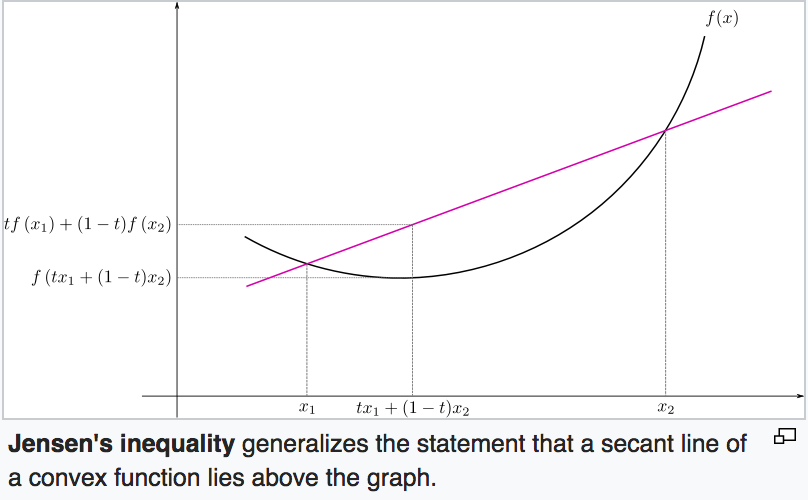# Expectation Maximization (EM)

## Summary

Expectation Maximization (EM) is an algorithm for obtaining the probability of some hidden random variable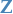given some observations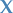.

It is a two-step algorithm, which are repeated in order until convergence:

1. Expectation-step: Choose some probability distribution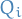,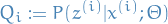1

which gives us a (tight) lower-bound for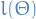for the curren estimate of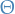2. Maximization-step: optimize the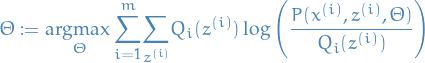2

Or rather, following the notation of Wikipedia:

1. Expectation step (E step): Calculate (or obtain an expression for) the expected value of the (complete) log likelihood function, with respect to the conditional distribution ofgivenunder the current estimate of the parameters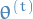: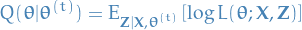3
2. Maximization step (M step): Find the parameter that maximizes this quantity: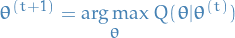4

## Notation

•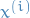is the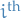observation
•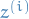is the rv. related to the•is a set of parameters for our model, which we want to estimate
•is the log-likelihood of the data given the estimated parameters•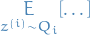means the expectation over the rv.using the probability distribution## Goal

• Have some model for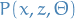• Observe only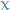• Objective is then to maximize: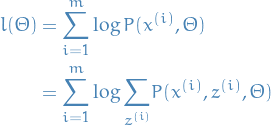5

i.e. maximize the log-likelihood over the parametersby marginalizing over the rvs..

## Idea

The idea behind the EM algorithm is to at each step construct a lower-bound for the log-likelihood using our estimate forfrom the previous step. In this sense, the EM algorithm is a type of inference known as variational inference.

The reason for doing this is that we can often find a closed-form optimal value for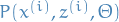, which is not likely to be the case if we simply tried to take the derivative of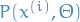set to zero and try to solve.

Finding the optimal parameters for the M-step is just to take the derivative of the M-step, set to zero and solve. It turns out that this is more often easier than directly doing the same for.

### Viewing it in the form coordinate descent

If we let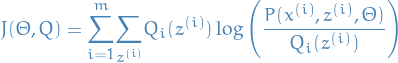6

then we know from Jensen's inequality that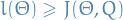, and so increasing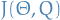at each step also monotonically increases the log-likelihood. It can be shown that this is then equivalent to using coordinate descent on.

## Derivation

If we take our objective defined above, we and simply multiply by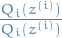, we get: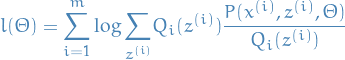7

Where we chooseto be s.t.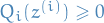and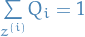, i.e.is a probability distribution.

We then observe that the last sum (within the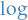) is actually the following expectation: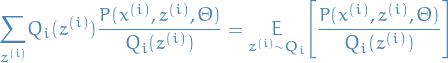8

Now using Jensen's inquality for a concave (because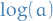is concave) function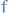, which tells us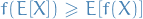: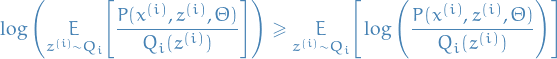9

Thus, we have constructed a lower-bound on the log-likelihood:10

Why? This would imply that the expectation above would also be constant. And since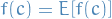is true for any constant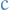, the inequality would be tight, i.e. be an equality, as wanted!

It's also equivalent of saying that we wantto be proportional to. Combining this with the fact that we wantto be a probability distribution, so we need to satisfy the proportionality and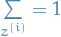. Then it turns out (makes sense, since we need it to sum to 1 and be prop to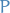) that we want: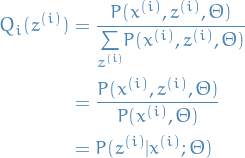11

Which gives us the E-step of the EM-algorithm:12

Giving us a (tight) lower-bound forfor the current estimate of.

The M-step is then to optimize that lower-bound wrt.:13

## Appendix A: Jensen's inquality

If

•is a convex function.
•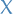is a rv.

Then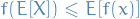.

Further, if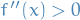(is strictly convex), then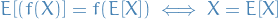"with probability 1".

•is a concave function
Then. This is the one we need for the derivation of EM-algorithm.
To get a visual intuition, here is a image from Wikipedia: